# High School Math : How to find the length of the side of of an acute / obtuse isosceles triangle

## Example Questions

### Example Question #19 : Triangles

A triangle has a perimeter of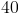inches with one side of lengthinches. If the remaining two sides have lengths in a ratio of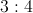, what is length of the shortest side of the triangle?The answer is.
Since we know that the permieter isinches and one side isinches, it can be determined that the remaining two sides must combine to be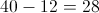inches. The ratio of the remaining two sides iswhich means 3 parts : 4 parts or 7 parts combined. We can then set up the equation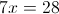, and divide both sides bywhich means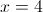. The ratio of the remaining side lengths then becomes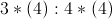or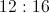. We now know the 3 side lengths are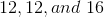.is the shortest side and thus the answer.